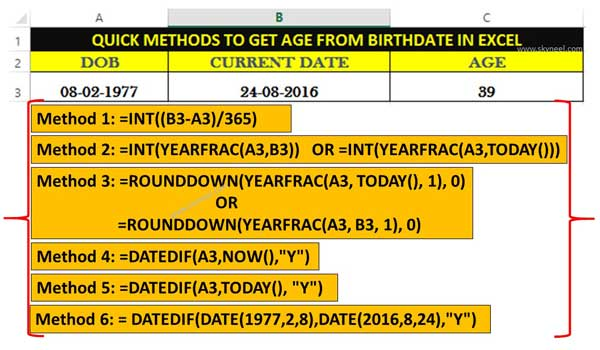# Quick methods to get age from birthdate in Microsoft Excel

If you need to calculate any person's age from his date of birth to current date then you can use given quick methods to get age from birthdate in Excel.

If you need to calculate any person’s age from his date of birth to current date then you can use different methods to get age from birthdate in Excel. There are lot of team members or employees working in the office and you can easily convert birthdate to age quickly using Excel. If you want to calculate your current age from your date of birth manually then you must take lot of time. Here we will discuss different methods which helps you to quickly calculate current age from birthdate in Microsoft Excel.

If you want to use manual calculation method to compute the age of any person one by one then you have to consume lot of time. But, if you don’t have enough time and also calculate age from birthdate then you have to take help different function in Excel. All we know Excel, is widely used application software around the world, which provide the quick result of complex calculations and many more. You can also apply a trick to calculate your age without using function in Excel.

## Quick methods to Get age from birthdate in Excel

Most of the person have to use one or two methods to calculate the age from birthdate in Excel. But, if this article we have to discuss several quick methods which helps you to get age from birthdate in Excel. If you want to calculate the age of any person’s then you have to take few given simple steps in this article.### Method 1: Using INT function

Step 1: Open an existing worksheet or create a new worksheet in Excel. Type your date of birth in MM/DD/YYYY format in cell A2 and current date in cell B2.

Step 2: Type the following given formula in cell C2 to get age from birthdate in Excel =INT((B2-A2)/365). Now, press enter you will get the year of any person’s age from his date of birth to current date.

### Method 2: Using INT with YEARFRAC function

Step 1: Open an existing worksheet or create a new worksheet in Excel. Type your date of birth in MM/DD/YYYY format in cell A2 and current date in cell B2.

Step 3: Type the following given formula in cell C2 to get age from birthdate in Excel =INT(YEARFRAC(A2,B2)). You can also type another formula =INT(YEARFRAC(A2,TODAY())) to calculate the age in Excel. Now, press enter you will get the year of any person’s age from his date of birth to current date.

### Method 3: Using ROUNDDOWN with YEARFRAC function

Step 1: Open an existing worksheet or create a new worksheet in Excel.  Type your date of birth in MM/DD/YYYY format in cell A2 and current date in cell B2.

Step 2: Type the following given formula in cell C2 to get age from birthdate in Excel =ROUNDDOWN(YEARFRAC(A2, TODAY(), 1), 0). You can also type another formula =ROUNDDOWN(YEARFRAC(A2, B2, 1), 0) to calculate the age in Excel. Now, press enter you will get the year of any person’s age from his date of birth to current date.

### Method 4: Using DATEDIF with NOW function

Step 1: Open an existing worksheet or create a new worksheet in Excel.

Step 2: Type your date of birth in MM/DD/YYYY format in cell A2 and current date in cell B2.

Step 3: Type the following given formula in cell C2 to get age from birthdate in Excel =DATEDIF(A2,NOW(),”Y”). Now, press enter you will get the year of any person’s age from his date of birth to current date.

### Method 5: Using DATEDIF with TODAY function

Step 1: First you have to create new worksheet or open an existing worksheet. Type your date of birth in MM/DD/YYYY format in cell A2 and current date in cell B2.

Step 2: Type the following given formula in cell C2 to get age from birthdate in Excel =DATEDIF(A2,TODAY(), “Y”). Now, press enter you will get the year of any person’s age from his date of birth to current date.

### Method 6: Using DATEDIF with DATE function

Step 1: Open an existing worksheet or create a new worksheet in Excel. Type your date of birth in MM/DD/YYYY format in cell A2 and current date in cell B2.

Step 2: Type the following given formula in cell C2 to get age from birthdate in Excel = DATEDIF(DATE(1977,2,8),DATE(2016,8,24),”Y”). Now, press enter you will get the year of any person’s age from his date of birth to current date.

I hope after reading this article you can easily understand quick methods to get age from birthdate in Excel. You have to quickly convert any person’s date of birth in to the age using the given functions in Excel. If you have any query regarding this guide then please write us in the comment box. Thanks to all.